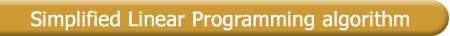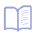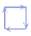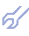Many ill-defined objects in macroeconomy, ecology, manufacturing etc. can be described accurately enough by static algebraic or by difference equations (with one or two delayed arguments only). This peculiarity makes it possible to apply linear programming for normative forecasting (after "what-if" scenario) and control optimization of averaged variables. Mathematical programming, linear or non-linear, can be used for optimization to find optimal values of input variables for each set of output variables. The most difficult problem is to determine necessary constrains of polygonal optimization area. Equations, describing constrains and goal functions, can be obtained by GMDH algorithm . For application in complex problems, the linear programming algorithm is to be revised and modified in following directions [7,21]: Instead of one scalar variable the vector of goal function is to be used; The procedure of tops polygonal area of constrains sorting-out is simplified. Modifications in linear programming algorithm are following: Set of variables given in the data sampling, is divided to two parts of input and output variables ( X = x1,x2,...,xM , Y = y1,y2,...,yL). Subset of output variables contains all of them, the reference optimal values of which, are known. The problem is to find corresponding optimal values of input variables; Each variable can be presented by its components. Therefore, we always can simplify calculations by making M=L. Algorithm is developed for this case only. First step in algorithm is calculation of differences Ri = yi - yiopt , i = 1,2,..,L Second step is obtaining of models of constrains by Combinatorial GMDH algorithm: Ri = fi (x1,x2,...,xM) or Ri = fi (x1,x2,...,xM, y1,y2,...,yi-1,yi+1,...,yL ) Third step is system of constrains equations solution Ri = 0. We obtain such way the solution of the problem x1opt x2opt ... x3opt In the case, when object has inertia is necessary to include as arguments into models of constrains the delayed values of input variables. Instead of one instant we shall have two-instant models of constrains Ri = fi (x1(k),...,xM(k) x1(k-1)...xM(k-1) x1(k-2)...xM(k-2)) Number of delayed argument, taking into account is to be slowly increased, until the accuracy criterion RR decreases. Two-instant or three-instant models of constrains are difference equations. They can be used for step-by-step forecasting procedure. Such forecast gives the answer to question, how the input variables will change in time if the output variables will be kept on optimal values (so called "normative forecasting"). Example of optimization of the world dynamics by multivariable linear programming is given here. The system of equations used in linear programming can be considered as the first layer of neural network with active neurons. If the error criterion is not small enough the next layers of neuronet can be constructed to increase accuracy of normative forecast and computer control. The use of optimal non-physical models for specification of constrains in linear programing and self-organization of twice-multilayered neuronet are two consistent steps in increase of accuracy recommended for ill-defined object control. Examples of use of Simplified Linear Programming algorithm should be used for computer advisers construction, normative forecasting (after "what-if-then" scenario) and control optimization of averaged variable.